This book is archived and will be removed July 6, 2022. Please use the updated version.

Long-Term Liabilities

# 79 Compute Amortization of Long-Term Liabilities Using the Effective-Interest Method

In our discussion of long-term debt amortization, we will examine both notes payable and bonds. While they have some structural differences, they are similar in the creation of their amortization documentation.

### Pricing of Long-Term Notes Payable

When a consumer borrows money, she can expect to not only repay the amount borrowed, but also to pay interest on the amount borrowed. When she makes periodic loan payments that pay back the principal and interest over time with payments of equal amounts, these are considered fully amortized notes. In these timed payments, part of what she pays is interest. The amount borrowed that is still due is often called the principal. After she has made her final payment, she no longer owes anything, and the loan is fully repaid, or amortized. Amortization is the process of separating the principal and interest in the loan payments over the life of a loan. A fully amortized loan is fully paid by the end of the maturity period.

In the following example, assume that the borrower acquired a five-year, \$10,000 loan from a bank. She will repay the loan with five equal payments at the end of the year for the next five years. The bank’s required interest rate is an annual rate of 12%.

Interest rates are typically quoted in annual terms. Since her interest rate is 12% a year, the borrower must pay 12% interest each year on the principal that she owes. As stated above, these are equal annual payments, and each payment is first applied to any applicable interest expenses, with the remaining funds reducing the principal balance of the loan.

After each payment in a fully amortizing loan, the principal is reduced, which means that since the five payment amounts are equal, the portion allocated to interest is reduced each year, and the amount allocated to principal reduction increases an equal amount.

We can use an amortization table, or schedule, prepared using Microsoft Excel or other financial software, to show the loan balance for the duration of the loan. An amortization table calculates the allocation of interest and principal for each payment and is used by accountants to make journal entries. These journal entrieswill be discussed later in this chapter.

The first step in preparing an amortization table is to determine the annual loan payment. The \$10,000 loan amount is the value today and, in financial terms, is called the present value (PV). Since repayment will be in a series of five equal payments, it is an annuity. Look up the PV from an annuity table for 5 periods and 12% interest. The factor is 3.605. Dividing the principal, \$10,000, by the factor 3.605 gives us \$2,773.93, which is the amount of each yearly payment. For the rest of the chapter, we will provide the necessary data, such as bond prices and payment amounts; you will not need to use the present value tables.

When the first payment is made, part of it is interest and part is principal. To determine the amount of the payment that is interest, multiply the principal by the interest rate (\$10,000 × 0.12), which gives us \$1,200. This is the amount of interest charged that year. The payment itself (\$2,773.93) is larger than the interest owed for that period of time, so the remainder of the payment is applied against the principal.

(Figure) shows an amortization table for this \$10,000 loan, over five years at 12% annual interest. Assume that the final payment will be \$2,774.99 in order to eliminate the potential rounding error of \$1.06.

Amortization Table. An amortization table shows how payments are applied to interest in principal for the life of the loan. (attribution: Copyright Rice University, OpenStax, under CC BY-NC-SA 4.0 license)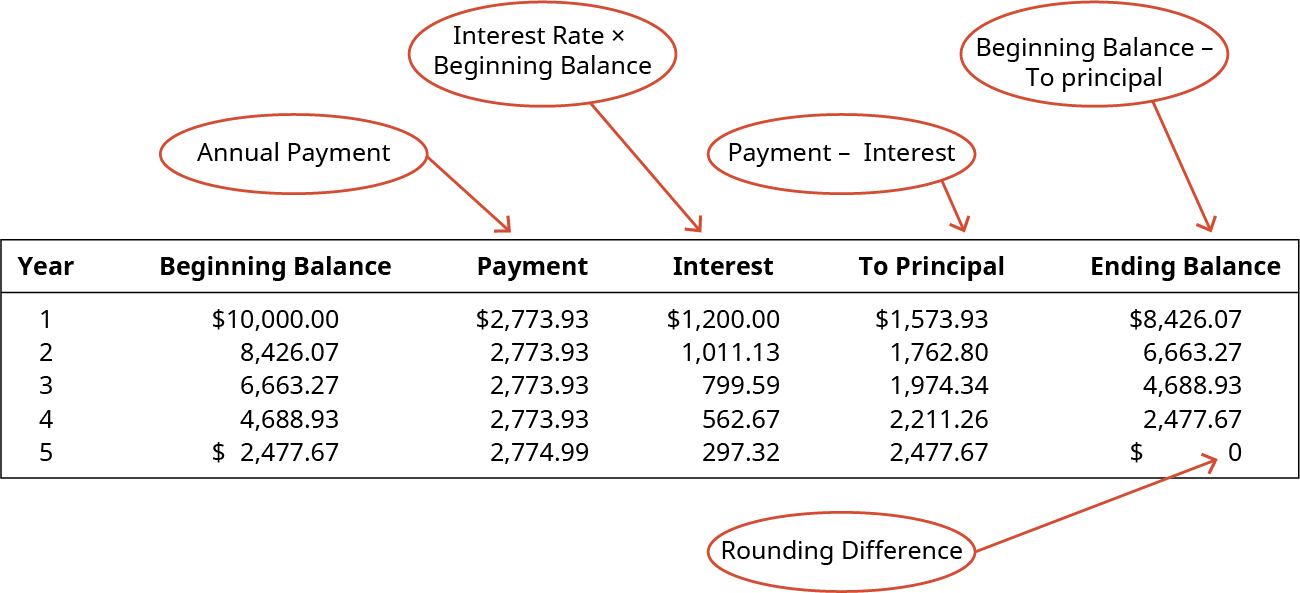You want to borrow \$100,000 for five years when the interest rate is 5%. You will make yearly payments of \$23,097.48 for 5 years. Fill in the blanks in the amortization table below. Assume that the loan was created on January 1, 2018 and totally repaid by December 31, 2022, after five equal, annual payments.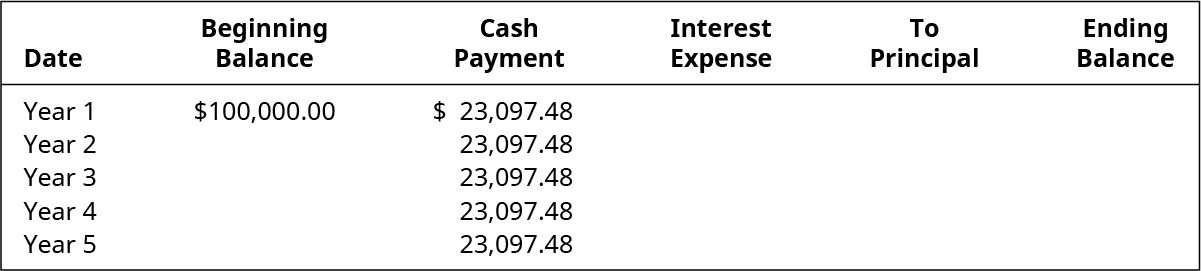Solution

Multiply the \$100,000 by the 5% interest rate and \$5,000 is the amount of interest you owe for year 1. Subtract the interest from the payment of \$23,097.48 to find \$18,097.48 is applied toward the principal (\$100,000), leaving \$81,902.52 as the ending balance. In year 2, \$81,902.52 is charged 5% interest (\$4,095.13), but the rest of the 23,097.48 payment goes toward the loan balance. Follow the same process for years 3 through 5.### Bonds Payable

As you’ve learned, each time a company issues an interest payment to bondholders, amortization of the discount or premium, if one exists, impacts the amount of interest expense that is recorded. Amortization of the discounts increases the amount of interest expense and premiums reduce the amount of interest expense. There are two methods used to amortize bond discounts or premiums: the effective-interest method and the straight-line method.

Our calculations have used what is known as the effective-interest method, a method that calculates interest expense based on the carrying value of the bond and the market interest rate. Generally accepted accounting principles (GAAP) require the use of the effective-interest method unless there is no significant difference between the effective-interest method and the straight-line method, a method that allocates the same amount of the bond discount or premium for each interest payment. The effective interest amortization method is more accurate than the straight-line method. International Financial Reporting Standards (IFRS) require the use of the effective-interest method, with no exceptions.

The straight-line method doesn’t base its calculation of amortization for a period base on a changing carrying value like the effective-interest method does; instead, it allocates the same amount of premium or discount amortation for each of the bond’s payment periods.

For example, assume that \$500,000 in bonds were issued at a price of \$540,000 on January 1, 2019, with the first annual interest payment to be made on December 31, 2019. Assume that the stated interest rate is 10% and the bond has a four-year life. If the straight-line method is used to amortize the \$40,000 premium, you would divide the premium of \$40,000 by the number of payments, in this case four, giving a \$10,000 per year amortization of the premium. (Figure) shows the effects of the premium amortization after all of the 2019 transactions are considered. The net effect of creating the \$40,000 premium and writing off \$10,000 of it gives the company an interest expense of \$40,000 instead of \$50,000, since the \$50,000 expense is reduced by the \$10,000 premium write down at the end of the year.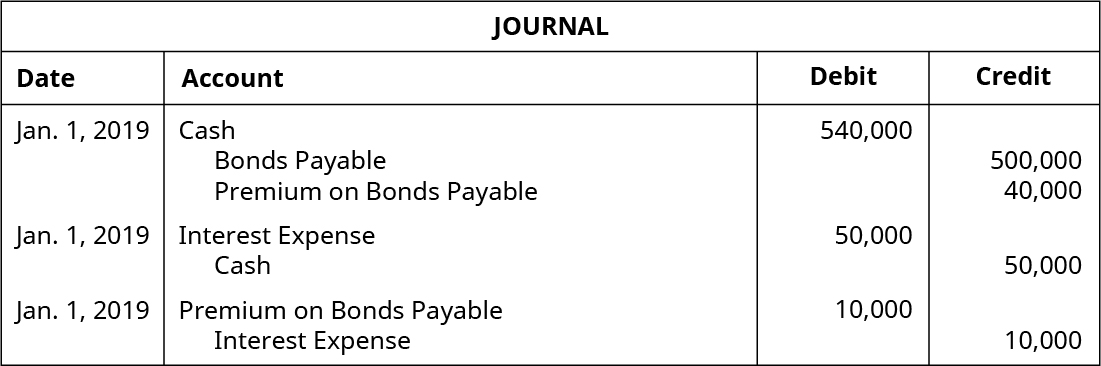#### Issued When Market Rate Equals Contract Rate

Assume a company issues a \$100,000 bond with a 5% stated rate when the market rate is also 5%. The bond was issued at par, meaning it sold for \$100,000. There was no premium or discount to amortize, so there is no application of the effective-interest method in this example.

The same company also issued a 5-year, \$100,000 bond with a stated rate of 5% when the market rate was 4%. This bond was issued at a premium, for \$104,460. The amount of the premium is \$4,460, which will be amortized over the life of the bond using the effective-interest method. This method of amortizing the interest expense associated with a bond is similar to the amortization of the note payable described earlier, in which the principal was separated from the interest payments using the interest rate times the principal.

Begin by assuming the company issued all the bonds on January 1 of year 1 and the first interest payment will be made on December 31 of year 1. The amortization table begins on January 1, year 1, with the carrying value of the bond: the face value of the bond plus the bond premium.

On December 31, year 1, the company will have to pay the bondholders \$5,000 (0.05 × \$100,000). The cash interest payment is the amount of interest the company must pay the bondholder. The company promised 5% when the market rate was 4% so it received more money. But the company is only paying interest on \$100,000—not on the full amount received. The difference in the sale price was a result of the difference in the interest rates so both rates are used to compute the true interest expense.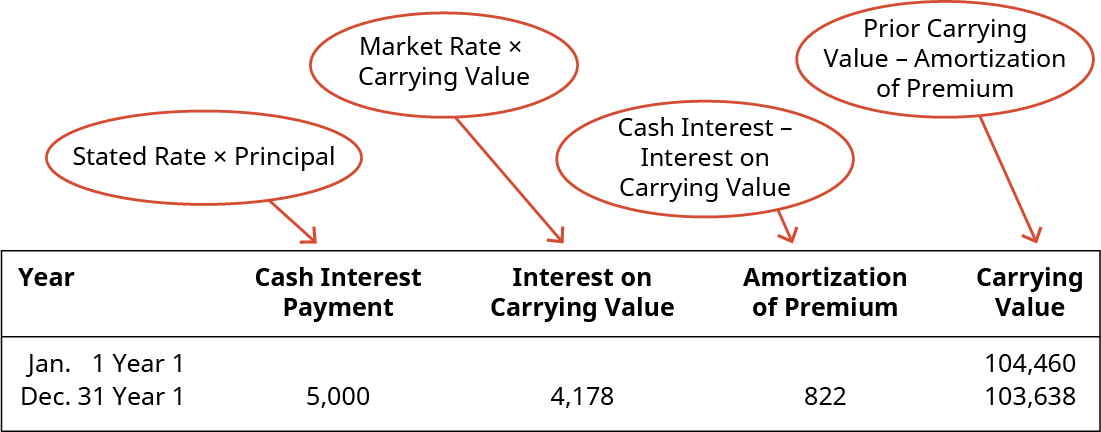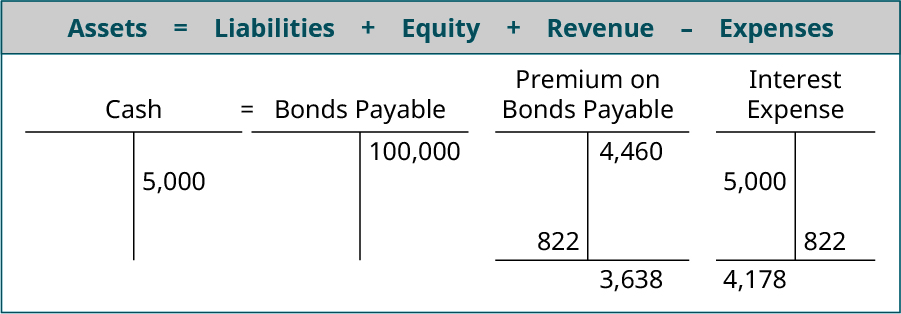The interest on the carrying value is the market rate of interest times the carrying value: 0.04 × \$104,460 = \$4,178. If the company had issued the bonds with a stated rate of 4%, and received \$104,460, it would be paying \$4,178 in interest. The difference between the cash interest payment and the interest on the carrying value is the amount to be amortized the first year. The complete amortization table for the bond is shown in (Figure). The table is necessary to provide the calculations needed for the adjusting journal entries.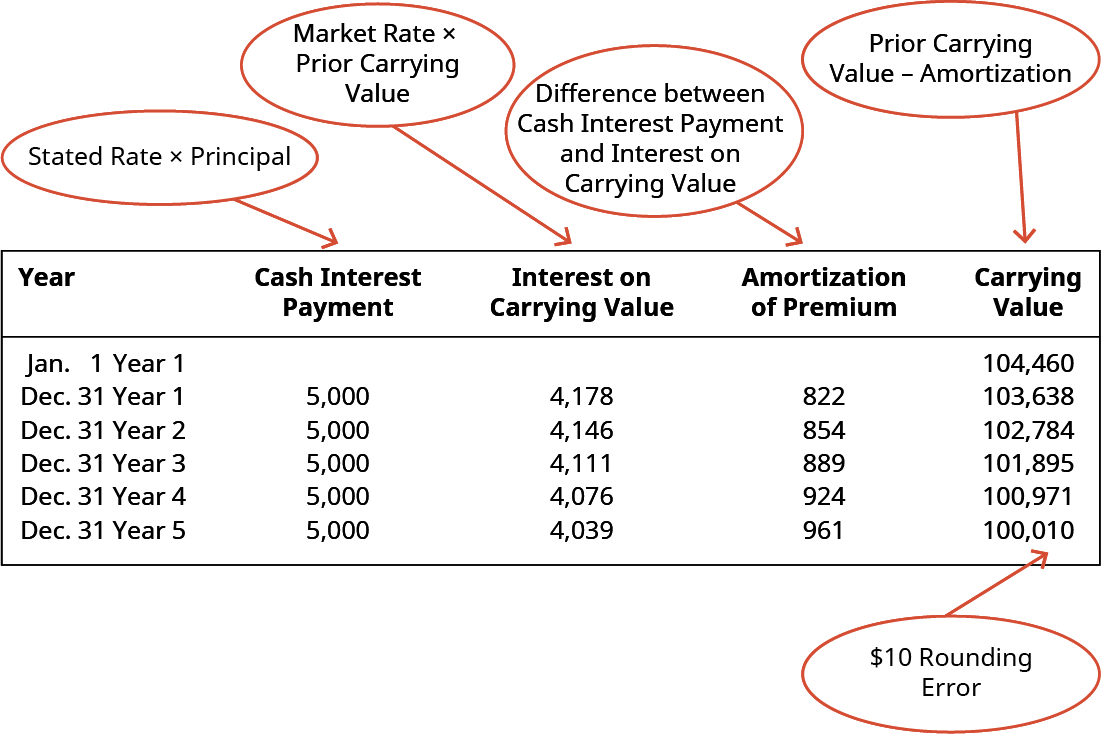#### Issued at a Discount

The company also issued \$100,000 of 5% bonds when the market rate was 7%. It received \$91,800 cash and recorded a Discount on Bonds Payable of \$8,200. This amount will need to be amortized over the 5-year life of the bonds. Using the same format for an amortization table, but having received \$91,800, interest payments are being made on \$100,000.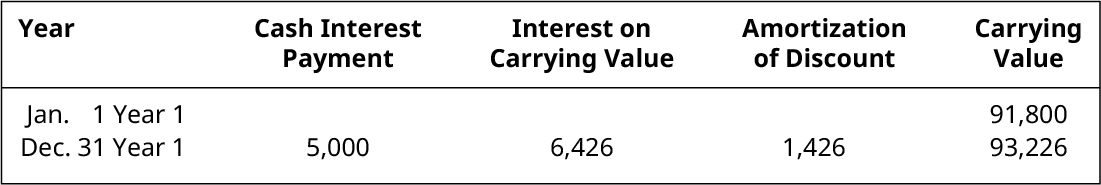The cash interest payment is still the stated rate times the principal. The interest on carrying value is still the market rate times the carrying value. The difference in the two interest amounts is used to amortize the discount, but now the amortization of discount amount is added to the carrying value.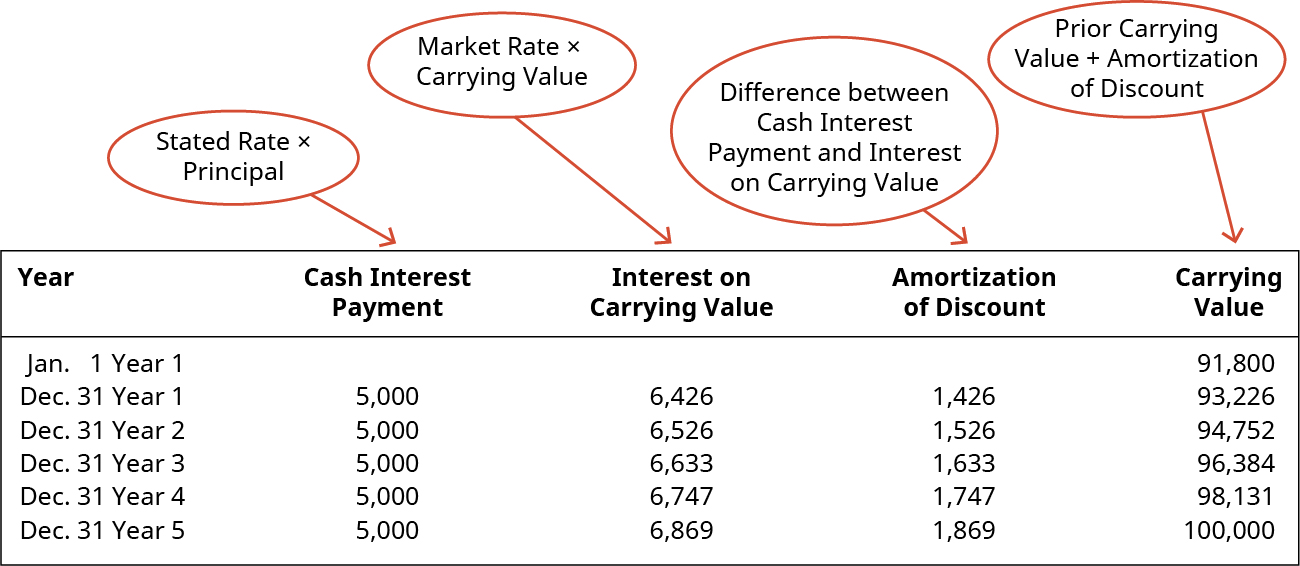(Figure) illustrates the relationship between rates whenever a premium or discount is created at bond issuance.

Stated Rate and Market Rate. When the stated rate is higher than the market rate, the bond is issued at a premium. When the stated rate is lower than the market rate, the bond is issued at a discount. (attribution: Copyright Rice University, OpenStax, under CC BY-NC-SA 4.0 license)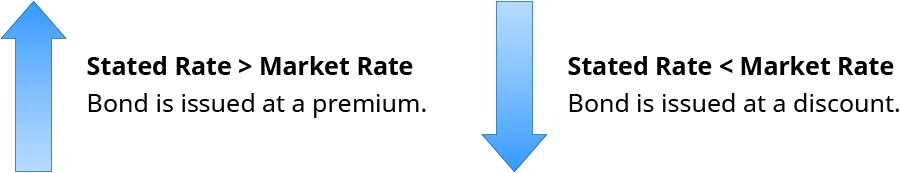Bond Ratings

Investors intending to purchase corporate bonds may find it overwhelming to decide which company would be the best to invest in. Investors are concerned with two primary factors: the return on the investment (meaning, the periodic interest payments) and the return of the investment (meaning, payment of the face value on the maturity date). While there are risks with any investment, attempting to maximize the return on the investment and maximizing the likelihood receiving the return of the investment would take a significant amount of time for the investor. To become informed and make a wise investment, the investor would have to spend many hours analyzing the financial statements of potential companies to invest in.

One resource investors find useful when screening investment opportunities is through the use of rating agencies. Rating agencies specialize in analyzing financial and other company information in order to assess and rate a company’s riskiness as an investment. A particularly useful website is Investopedia which highlights the rating system for three large rating agencies—Moody’s, Standard & Poor’s, and Fitch Ratings. The rating systems, shown below, are somewhat similar to academic grading scales, with rankings ranging from A (highest quality) to D (lowest quality):

Rating Agencies1
Credit Risk Moody’s Standard & Poor’s Fitch Ratings
Highest Quality Aaa AAA AAA
High Quality Aa1, Aa2, Aa3 AA+, AA, AA– AA+, AA, A–
Upper Medium A1, A2, A3 A+, A, A– A+, A, A–
Medium Baa1, Baa2, Baa3 BBB+, BBB, BBB– BBB+, BBB, BBB–
Not Investment Grade Ba1 BB+ BB+
Speculative Medium Ba2, Ba3 BB, BB– BB, BB–
Speculative Lower Grade B1, B2, B3 B+, B, B– B+, B, B–
Speculative Risky Caa1 CCC+ CCC
Speculative Poor Standing Caa2, Caa3 CCC, CCC–
No Payments / Bankruptcy Ca / C
In Default D DDD, DD, D

### Key Concepts and Summary

• The effective-interest method is a common method used to calculate the interest expense for a given interest payment.
• There is an inverse relationship between the price of a bond and the market interest rate.
• The carrying value of a bond sold at a discount will increase during the life of a bond until the maturity or face value is reached.
• The carrying value of a bond sold at a premium will decrease during the life of a bond until the maturity or face value is reached.
• The amount of cash to be paid, the interest expense, and the premium or discount amortization (when applicable) with each periodic payment are calculated based on an amortization table or schedule.

### Multiple Choice

(Figure)The effective-interest method of bond amortization finds the difference between the ________ times the ________ and the ________ times the ________.

1. stated interest rate, principal, stated interest rate, carrying value
2. stated interest rate, principal, market interest rate, carrying value
3. stated interest rate, carrying value, market interest rate, principal
4. market interest rate, carrying value, market interest rate, principal

(Figure)When a bond sells at a discount, the carrying value ________ after each amortization entry.

1. increases
2. decreases
3. stays the same
4. cannot be determined

A

(Figure)The International Financial Reporting Standards require the use of ________.

1. any method of amortization of bond premiums
2. the straight-line method of amortization of bond discounts
3. the effective-interest method of amortization of bond premiums and discounts
4. any method approved by US GAAP

(Figure)The cash interest payment a corporation makes to its bondholders is based on ________.

1. the market rate times the carrying value
2. the stated rate times the principal
3. the stated rate times the carrying value
4. the market rate times the principal

B

(Figure)Whirlie Inc. issued \$300,000 face value, 10% paid annually, 10-year bonds for \$319,251 when the market of interest was 9%. The company uses the effective-interest method of amortization. At the end of the year, the company will record ________.

1. a credit to cash for \$28,733
2. a debit to interest expense for \$31,267
3. a debit to Discount on Bonds Payable for \$1,267
4. a debit to Premium on Bonds Payable for \$1.267

### Questions

(Figure)An amortization table/schedule is created to compute the amount to be amortized each year. What are the four columns needed to prepare the table?

(Figure)In the amortization table, how is the amortization of discount of premium computed?

It is the difference between the cash interest payment and the interest on the carrying value.

(Figure)Does issuing a bond at a discount increase or decrease interest expense over the life of the bond?

(Figure)What kind of account is the Discount on Bonds Payable? What kind of account is the Premium on Bonds Payable?

The Discount on Bonds Payable is a contra liability account and reduces the associated liability. The Premium on Bonds Payable is a liability account and increases the associated liability.

(Figure)Why is the effective-interest method of amortization required under the International Financial Reporting Standards?

### Exercise Set A

(Figure)Diana Inc. issued \$100,000 of its 9%, 5-year bonds for \$96,149 when the market rate was 10%. The bonds pay interest semi-annually. Prepare an amortization table for the first three payments.

(Figure)Oak Branch Inc. issued \$700,000 of 5%, 10-year bonds when the market rate was 4%. They received \$757,243. Interest was paid semi-annually. Prepare an amortization table for the first three years of the bonds.

### Exercise Set B

(Figure)Haiku Inc. issued \$600,000 of 10-year bonds with a stated rate of 11% when the market rate was 12%. The bonds pay interest semi-annually. Prepare the first three years of an amortization schedule. Assume that the bonds were issued for \$565,710.

(Figure)Waldron Inc. issued \$400,000 bonds with a stated rate of 7% when the market rate was 5%. They are 3-year bonds with interest to be paid annually. Prepare a table to amortize the premium of the bonds. Assume that the bonds were issued for \$421,844.

### Problem Set A

(Figure)Eli Inc. issued \$100,000 of 8% annual, 5-year bonds for \$103,000. What is the total amount of interest expense over the life of the bonds?

(Figure)Evie Inc. issued 50 bonds with a \$1,000 face value, a five-year life, and a stated annual coupon of 6% for \$980 each. What is the total amount of interest expense over the life of the bonds?

### Problem Set B

(Figure)Starmount Inc. sold bonds with a \$50,000 face value, 12% interest, and 10-year term at \$48,000. What is the total amount of interest expense over the life of the bonds?

(Figure)Irving Inc. sold bonds with a \$50,000, 10% interest, and 10-year term at \$52,000. What is the total amount of interest expense over the life of the bonds?

### Thought Provokers

(Figure)Below is select information from two, independent companies.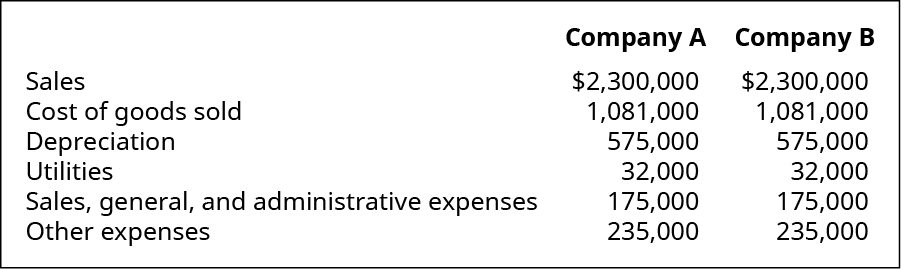• On January 1, Company A issued a 5-year \$1,500,000 bond with at 6% stated rate. Interest is paid semiannually and the bond was sold at 105.5055 to yield a market rate of 4.75%.
• On January 1, Company B sold \$1,500,000 of common stock and paid dividends of \$75,000.
1. Prepare an income statement for each company (ignore taxes)
2. Explain why the net income amounts are different, paying particular attention to the operational performance and financing performance of each company. (Hint: it may be helpful for you to create an amortization table).

### Footnotes

• 1 Michael Schmidt. “When to Trust Bond Rating Agencies.” Investopedia. September 29, 2018.
https://www.investopedia.com/articles/bonds/09/bond-rating-agencies.asp

### Glossary

amortization
allocation of the costs of intangible assets over their useful economic lives; also, process of separating the principal and interest in loan payments over the life of a loan
effective-interest method
method of calculating interest expense based on multiplying the carrying value of the bond by the market interest rate
fully amortized notes
periodic loan payments that pay back the principal and interest over time with payments of equal amounts
straight-line method
method of calculating interest expense that allocates the same amount of premium or discount amortation for each of the bond’s payment periods